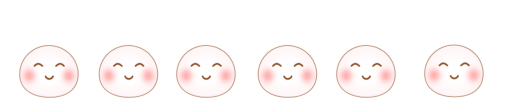40×130= 60×20=

50×220= 600×13=

520×10= 430×30=41+39-17=63（筐）

246+178=424（种）

40×130=5200

60×20=1200

50×220=11000

600×13=7800

520×10=5200

430×30=12900

1. 3.14×5×5=78.5（平方米）